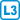## Long cycle

Show that each $$k$$-connected graph on at least $$2k$$ vertices contains a cycle of length at least $$2k$$.

• #### Hint

Use the fan theorem if the longest cycle is not long enough.

• #### Solution

If $$C$$ is the longest cycle and its length is less than $$2k$$, then we choose any vertex $$v$$ outside $$C$$. It follows from Menger’s theorem that between $$v$$ and $$C$$ exist $$k$$ vertex disjoint paths, some two of which lead to adjacent vertices of $$C$$ (according to Dirichlet’s principle). Instead of this edge, we extend the cycle $$C$$ through the paths and $$v$$.